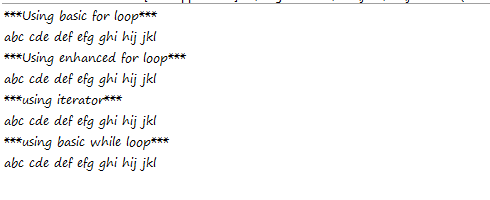### Different Ways to Traverse/Iterate/Loop a List In Java

In this post, i will show 4 basic ways to traverse/iterate/loop a list in java. They are

1. Using basic for loop.
2. Using enhanced for loop.
3. Using an Iterator.
4. Using an while loop.

consider you have a list of String objects. for example
`List<String> aList = Arrays.asList("abc","cde","def","efg","fgh","ghi","hij","ijk","jkl");`

1. Using Basic for Loop:

As Lists such as ArrayList, LinkedList are ordered in nature, you can use basic for loop, to iterate these type of collections.

The code is below.
```for(int i = 0, j = aList.size(); i < j; i++) {
System.out.print(aList.get(i)+" ");
}
```

2.Using Enhanced for Loop:

The enhanced for loop, iterates List and Set type of collections only.

The code in this approach is
```for(String item: aList) {
System.out.print(item+" ");
}
```

3.Using an Iterator with while Loop:

```Iterator aItr = aList.iterator();

while(aItr.hasNext()) {
System.out.print(aItr.next()+" ");
}
```

4.Using a basic while Loop:

what ever you can write using for loop, you can convert it into while loop. The below code snippet is similar to way 1.

```int i = 0, j = aList.size();
while(i < j) {
System.out.print(aList.get(i)+" ");
i++;
}
```

The complete code with all the approaches is
```package com.speakingcs.collections;

import java.util.Arrays;
import java.util.Iterator;
import java.util.List;

public class TraverseList {

public static void main(String[] args) {

TraverseList tl = new TraverseList();
tl.traverse();
}

private void traverse() {

List aList = Arrays.asList("abc","cde","def","efg","ghi","hij","jkl");

// using basic for looop

System.out.println("***Using basic for loop***");
for(int i = 0, j = aList.size(); i < j; i++) {
System.out.print(aList.get(i)+" ");
}

// using enhanced for loop
System.out.println("\n***Using enhanced for loop***");

for(String item: aList) {
System.out.print(item+" ");
}

// using iterator with while loop
System.out.println("\n***using iterator***");

Iterator aItr = aList.iterator();

while(aItr.hasNext()) {
System.out.print(aItr.next()+" ");
}

// using basic while loop

System.out.println("\n***using basic while loop***");

int i = 0, j = aList.size();
while(i < j) {
System.out.print(aList.get(i)+" ");
i++;
}
}

}
```

The Output is